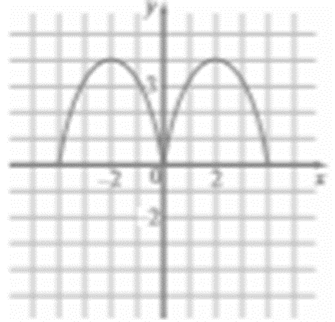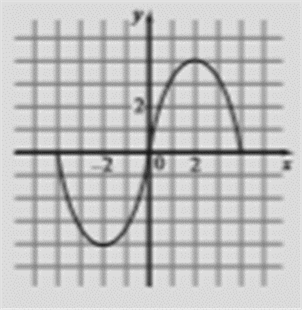# To graph the function for x &lt; 0 to make even function.### Precalculus: Mathematics for Calcu...

6th Edition
Stewart + 5 others
Publisher: Cengage Learning
ISBN: 9780840068071### Precalculus: Mathematics for Calcu...

6th Edition
Stewart + 5 others
Publisher: Cengage Learning
ISBN: 9780840068071

#### Solutions

Chapter 2.5, Problem 83E

a.

To determine

## To graph the function for x<0 to make even function.

Expert Solution

### Explanation of Solution

Given information:

The function is defined for x0 ,Graph :

the graph obtained as:Interpretation: from the above graph it is observed that when the graph defined for x0 , the graph for x<0 to make an even function plot the symmetric graph about y -axis.

b.

To determine

Expert Solution

### Explanation of Solution

Given information:

The function is defined for x0 ,Graph :

The graph obtained as:Interpretation: from the above graph it is observed that when the graph defined for x0 , the graph for x<0 to make an odd function plot the symmetric graph about x -axis

### Have a homework question?

Subscribe to bartleby learn! Ask subject matter experts 30 homework questions each month. Plus, you’ll have access to millions of step-by-step textbook answers!